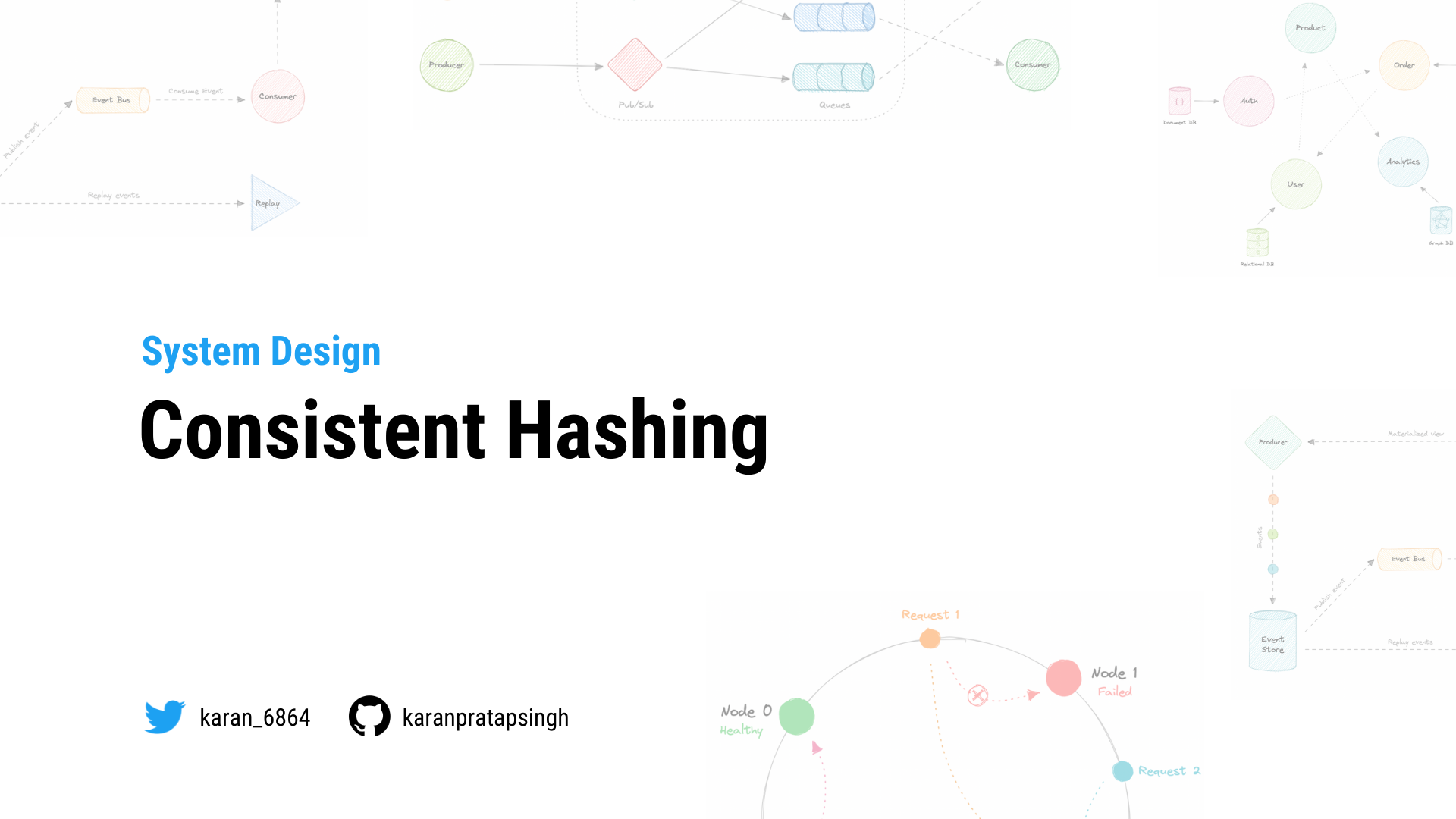# Consistent Hashing

Authors
•Name
Karan Pratap Singh
@karan_6864Let's first understand the problem we're trying to solve.

## Why do we need this?

In traditional hashing-based distribution methods, we use a hash function to hash our partition keys (i.e. request ID or IP). Then if we use the modulo against the total number of nodes (server or databases). This will give us the node where we want to route our request.\begin{align*} & Hash(key_1) \to H_1 \bmod N = Node_0 \\ & Hash(key_2) \to H_2 \bmod N = Node_1 \\ & Hash(key_3) \to H_3 \bmod N = Node_2 \\ & ... \\ & Hash(key_n) \to H_n \bmod N = Node_{n-1} \end{align*}

Where,

key: Request ID or IP.

H: Hash function result.

N: Total number of nodes.

Node: The node where the request will be routed.

The problem with this is if we add or remove a node, it will cause N to change, meaning our mapping strategy will break as the same requests will now map to a different server. As a consequence, the majority of requests will need to be redistributed which is very inefficient.

We want to uniformly distribute requests among different nodes such that we should be able to add or remove nodes with minimal effort. Hence, we need a distribution scheme that does not depend directly on the number of nodes (or servers), so that, when adding or removing nodes, the number of keys that need to be relocated is minimized.

Consistent hashing solves this horizontal scalability problem by ensuring that every time we scale up or down, we do not have to re-arrange all the keys or touch all the servers.

Now that we understand the problem, let's discuss consistent hashing in detail.

## How does it work

Consistent Hashing is a distributed hashing scheme that operates independently of the number of nodes in a distributed hash table by assigning them a position on an abstract circle, or hash ring. This allows servers and objects to scale without affecting the overall system.Using consistent hashing, only K/N data would require re-distributing.

$R = K/N$

Where,

R: Data that would require re-distribution.

K: Number of partition keys.

N: Number of nodes.

The output of the hash function is a range let's say 0...m-1 which we can represent on our hash ring. We hash the requests and distribute them on the ring depending on what the output was. Similarly, we also hash the node and distribute them on the same ring as well.

\begin{align*} & Hash(key_1) = P_1 \\ & Hash(key_2) = P_2 \\ & Hash(key_3) = P_3 \\ & ... \\ & Hash(key_n) = P_{m-1} \end{align*}

Where,

key: Request/Node ID or IP.

P: Position on the hash ring.

m: Total range of the hash ring.

Now, when the request comes in we can simply route it to the closest node in a clockwise (can be counterclockwise as well) manner. This means that if a new node is added or removed, we can use the nearest node and only a fraction of the requests need to be re-routed.

In theory, consistent hashing should distribute the load evenly however it doesn't happen in practice. Usually, the load distribution is uneven and one server may end up handling the majority of the request becoming a hotspot, essentially a bottleneck for the system. We can fix this by adding extra nodes but that can be expensive.

Let's see how we can address these issues.

## Virtual Nodes

In order to ensure a more evenly distributed load, we can introduce the idea of a virtual node, sometimes also referred to as a VNode.

Instead of assigning a single position to a node, the hash range is divided into multiple smaller ranges, and each physical node is assigned several of these smaller ranges. Each of these subranges is considered a VNode. Hence, virtual nodes are basically existing physical nodes mapped multiple times across the hash ring to minimize changes to a node's assigned range.For this, we can use k number of hash functions.

\begin{align*} & Hash_1(key_1) = P_1 \\ & Hash_2(key_2) = P_2 \\ & Hash_3(key_3) = P_3 \\ & . . . \\ & Hash_k(key_n) = P_{m-1} \end{align*}

Where,

key: Request/Node ID or IP.

k: Number of hash functions.

P: Position on the hash ring.

m: Total range of the hash ring.

As VNodes help spread the load more evenly across the physical nodes on the cluster by diving the hash ranges into smaller subranges, this speeds up the re-balancing process after adding or removing nodes. This also helps us reduce the probability of hotspots.

## Data Replication

To ensure high availability and durability, consistent hashing replicates each data item on multiple N nodes in the system where the value N is equivalent to the replication factor.

The replication factor is the number of nodes that will receive the copy of the same data. In eventually consistent systems, this is done asynchronously.

Let's look at some advantages of consistent hashing:

• Makes rapid scaling up and down more predictable.
• Facilitates partitioning and replication across nodes.
• Enables scalability and availability.
• Reduces hotspots.